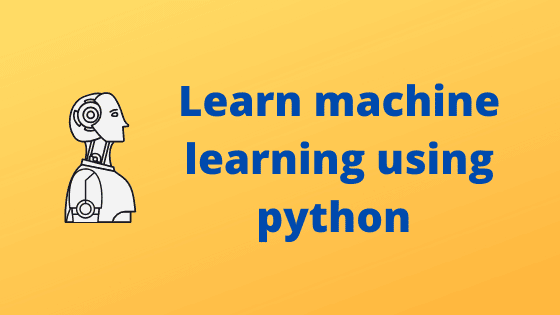In this post am going to share with you the idea that you need to follow to learn machine learning using python programming and in order to become a machine learning engineer.learn machine learning

so if you are seeking the answer to question that how you learn machine learning and become a machine learning engineer. then you are in the right place.
let's read the post from start to end to get an idea that how you can learn ML.

before going ahead you need to clear first that what is machine learning. because without knowing that what is machine learning you probably get the wrong idea about it.
so I have a fresh post on the topic of what is machine learning. so first read this post the link is given below.
What is machine learning?

Now let's come to our main topic. one of the most important things we all need to consider that for learning anything we need the presence of mind and full dedication.

## How to learn Machine learning using python programming?

For learning machine learning using python programming you need to follow these steps.

1. Learn basic to advance python.
2. Learn about the Numpy package.
3. Learn about the Pandas package.
4. Learn statistics.
5. Learn about matplotlib.
6. Learn about the seaborn library.
7. Explore data sets.
8. Understand Machine learning algorithms.
9. Learn about Hyperparameter tuning.
10. Learn about the Deployment technique

### Learn Python

Python is a scripting programming language. it has very powerful libraries for machine learning. so it fits very well as a programming language when we need a programming language for learning machine learning. so, first of all, you need to learn about python from basic to advance. because before learning machine learning you need to have at least one programming language knowledge.

### Learn about Numpy

Numpy is an inbuild python package. which handles the arrays with one and two dimensional. these arrays used to store the data for training machine learning models. so you need to learn about arrays and operations used on arrays using NumPy.

### Learn about Pandas

Pandas is an inbuild python package. it is used to manipulating data. it has data series and data frames used to manipulate data. so in pandas, you need to learn about data series and data frames. and different operations on data frames and data series.

### Learn statistics.

In machine learning, we always need statistics to train a model. so you need to learn statistics. this will give you an idea about how to calculate mean, median, variance, and mode. statistics is very important for learning machine learning. because machine learning depends on mathematics.

### Learn about Matplotlib

you need to learn about the Matplotlib library. it has a great API for plotting data in different forms. so using matplotlib you can plot a graph of data.

### Learn about Seaborn

Seaborn library used for data visualization. using seaborn we can draw a statical graph. so after learning matplotlib, you need to learn about the seaborn library.

### Explore the data set.

Exploring the data sets means trying to find out the maximum data about the given data set. it is very important to explore the data set when we use our data to train our model.

### Learn about Machine learning algorithms

There are hundreds of algorithms available for the machine learning model. so you need to learn about the algorithms used in machine learning. this will give you the idea of how to train a machine learning model. and which algorithm is fit for a particular machine learning model.

### Learn about Hyperparameter tuning.

after learning about machine learning algorithms you need to learn about hyperparameter tuning. it is nothing but how we can use algorithms on different inputs. so you need to understand how an algorithm works on different inputs.

### Learn about the Deployment technique.

after learning all these things that how to train a model using algorithms on different inputs. now you need to learn about deployment techniques. like how to deploy a machine learning model on cloud-like on AWS, on google cloud, on the server.

these are the thing which you need to learn to become a machine learning engineer using python programming. all these are essential steps that you need to follow to learn machine learning.

today is world machine learning is high in demand and a high-paying job.